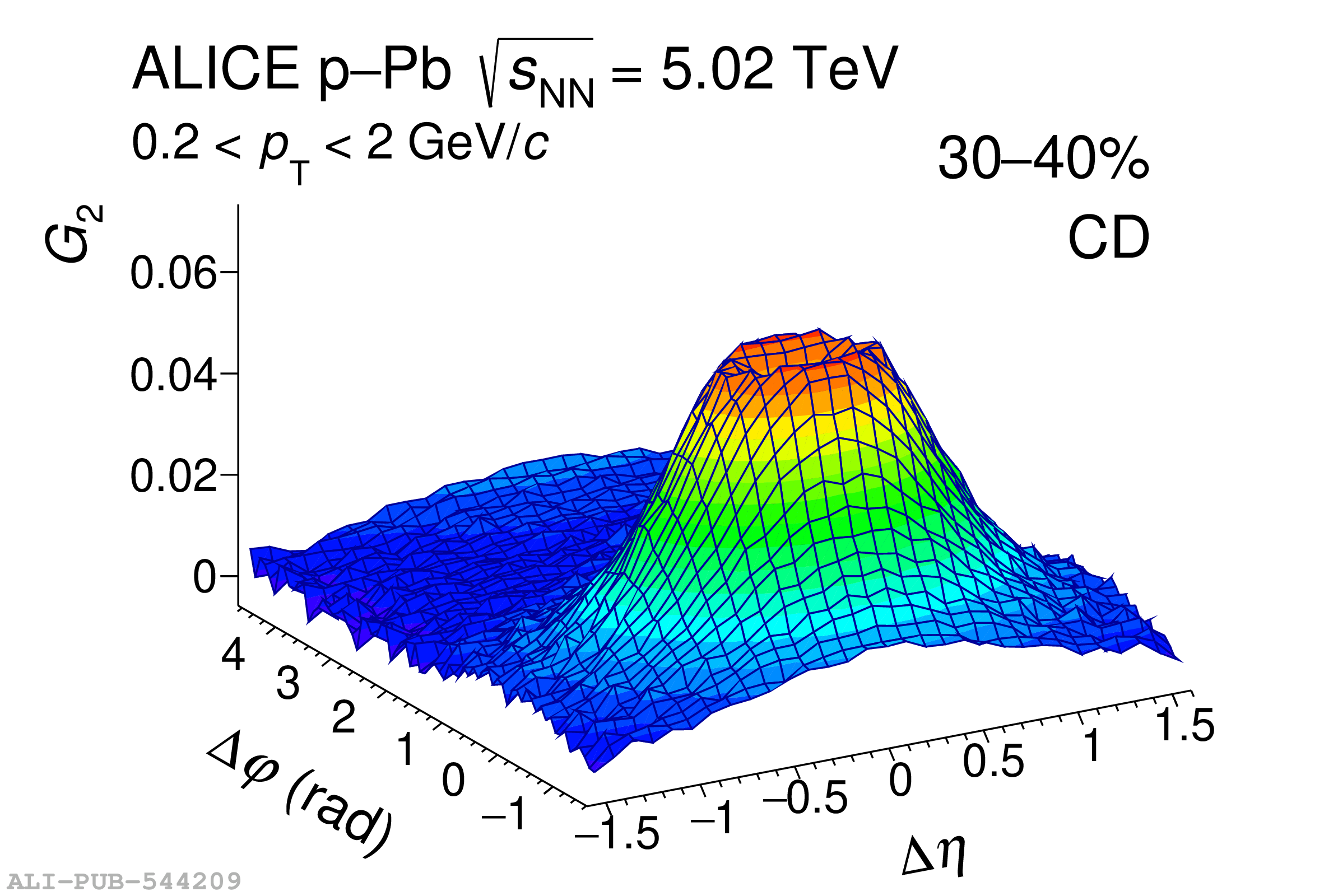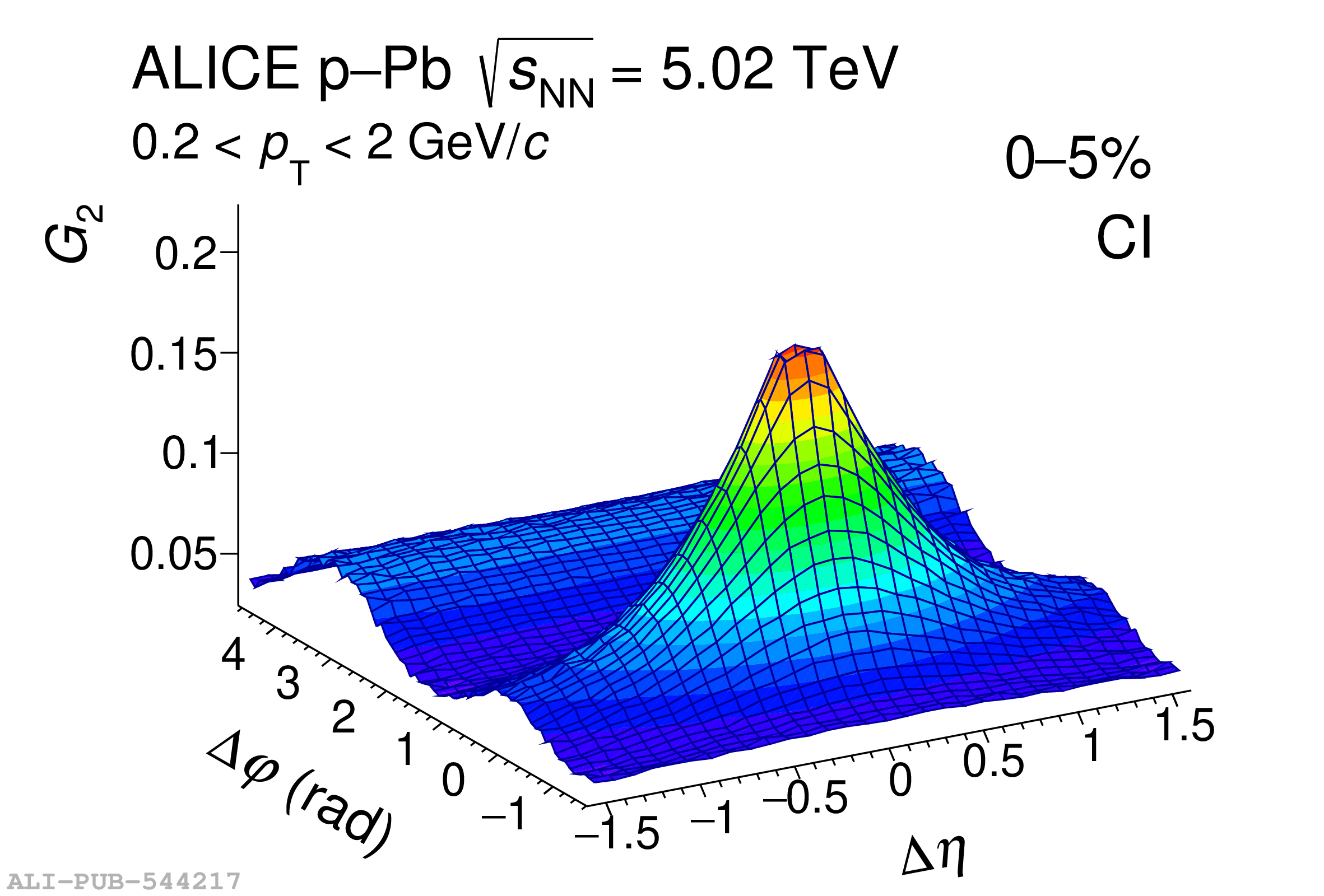# Figure 2

 Two-particle transverse momentum correlations $G_{2}^{\rm CD}$ (top) and $G_{2}^{\rm CI}$ (bottom) for the largest (left), medium (centre), and lowest (right) charged particle multiplicity classes in p--Pb collisions at $\sqrt{s_{\rm NN}}=5.02\;\text{TeV}$. The correlator values are not shown in the intervals $|\Delta\eta|<0.1$ and $|\Delta\varphi|<0.09$, which are affected by track merging effects (see text for details).